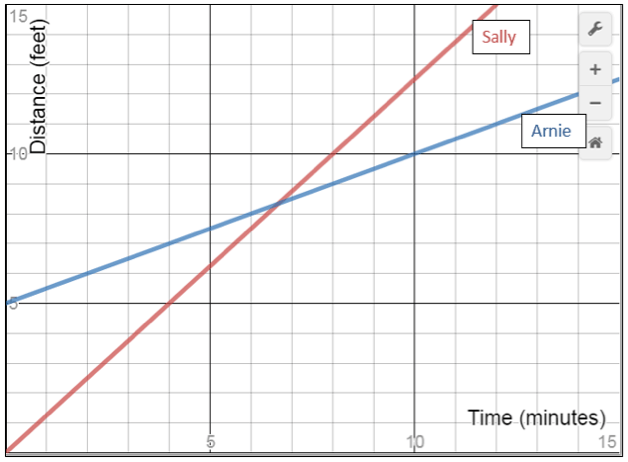# LINR 3 | Lesson 1 | Explore (System of Equations)# System of Equations

Sally the Spider and Arnie the Ant had a race depicted in the graph below. Graph is created using Desmos.com.What do you notice? What do you wonder?

Write everything you notice about the situation and graph, as well as any mathematical questions you have or could ask about this situation.

Some ideas you may consider

Now that you have had time to explore the above scenario, answer the following questions:

• Suppose Sally won. What does this tell you? What more do you need to know?
• Suppose the race ended at 5 minutes. What does this tell you? 10 minutes?
• If the race ended at 8 feet, what does that tell you? 10 feet?